# Bomber

The aircraft flies at an altitude of 4100 m above the ground at speed 777 km/h. At what horizontal distance from the point B should be release any body from the aircraft body to fall into point B? (g = 9.81 m/s2)

x =  6240 m

### Step-by-step explanation: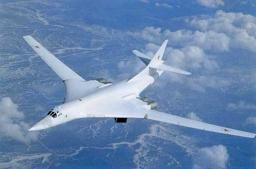Did you find an error or inaccuracy? Feel free to write us. Thank you!Tips to related online calculators
Do you want to convert length units?
Do you want to convert velocity (speed) units?
Do you want to convert time units like minutes to seconds?

## Related math problems and questions:

• Bomber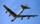Bomber flies 10 km at 600 km/h. At what horizontal distance from the target, must pilot drop the bomb to hit the target? Don't care about air resistance and consider the gravitational acceleration g=9.81 m/s2.
• The bomber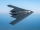From what distance in front of the target must a parachute load be dropped from an aircraft flying at an altitude of 1260 m if it slopes at a speed of 5.6 m/s and at the same time is carried in the direction of movement at a speed of 12 m/s. What is the d
• G forcesCalculate deceleration of car (as multiple of gravitational acceleration g = 9.81 m/s2) occurs when a vehicle in a frontal collision slows down uniformly from a speed 111 km/h to 0 km/h in 1.2 meters trajectory.
• Free fall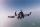Lloyd fall from height 7 m. Calculate the speed he hit the ground when falling with acceleration g = 9.81 m/s2
• ParatrooperAfter the parachute is opened, the paratrooper drops to the ground at a constant speed of 2 m/s, with the sidewinding at a steady speed of 1.5 m/s. Find: a) the magnitude of its resulting velocity concerning the ground, b) the distance of his land from a
• CollisionThe two bodies, whose initial distance is 240 m, move evenly against each other consistently. The first body has an initial velocity of 4 m/s and an acceleration of 3 m/s2, the second body has an initial speed of 6 m/s and an acceleration of 2 m/s2. Fin
• Two aircraftTwo planes fly to the airport. At some point, the first airplane is away from the airport 98 km and the second 138 km. The first aircraft flies at an average speed of 420 km/h, the second average speed is 360 km/h, while the tracks of both planes are perp
• An accelerationThe car goes on a straight road at a speed of 72 km/h. At some point, the driver starts to brake and stops the car in 5 seconds. Find: (a) the acceleration during braking (b) the distance traveled during braking.
• Propeller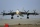The aircraft propeller rotates at an angular speed of 200 rad/s. A) What is the speed at the tip of the propeller if its distance from the axis of rotation is 1.5 m? B) What path does the aircraft travel during one revolution of the propeller at a speed o
• GravitationFrom the top of the 80m high tower, the body is thrown horizontally with an initial speed of 15 m/s. At what time and at what distance from the foot of the tower does the body hit the horizontal surface of the Earth? (use g = 10 ms-2)
• Free fall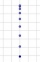For how long and at what speed does the body fall to the ground during a free fall from a height of 35 m?
• CarAt what horizontal distance reaches the car weight m = 753 kg speed v = 74 km/h when the car engine develops a tensile force F = 3061 N. (Neglect resistance of the environment.)
• Train speed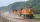The train speed is decreased during 50 sec from 72 km/h to 36 km/h. Assuming that the train movement is equally slowing, find the the acceleration and the distance that it travels at.
• AccelerationThe car accelerates at rate 0.5m/s2. How long travels 400 meters and what will be its speed?
• Forth and back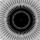Car drive from point A to point B at speed 78 km/h and back at 82 km/h. If went there and back at speed 81 km/h journey would take five minutes less. What is the distance between points A and B?
• AbyssThe stone fell into the abyss: 2 seconds after we heard hitting bottom. How deep is the abyss (neglecting air resistance)? (gravitational acceleration g = 9.81 m/s2 and the speed of sound in air v = 343 m/s)
• DriverThe car driver drives at a speed of 100 km/h, saw the obstacle, and began to brake with a slowing of 5 m/s². What is the length of the pathway to stopping the car when the driver has registered the obstacle with a delay of 0.7 s?